# What mass of water can be heated from 5.0 °C to 52 °C with 130 cal...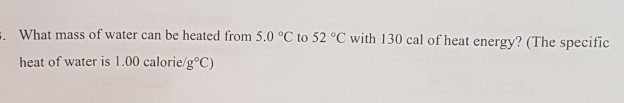What mass of water can be heated from 5.0 °C to 52 °C with 130 cal of heat energy? (The specific heat of water is 1.00 calorie/gºC)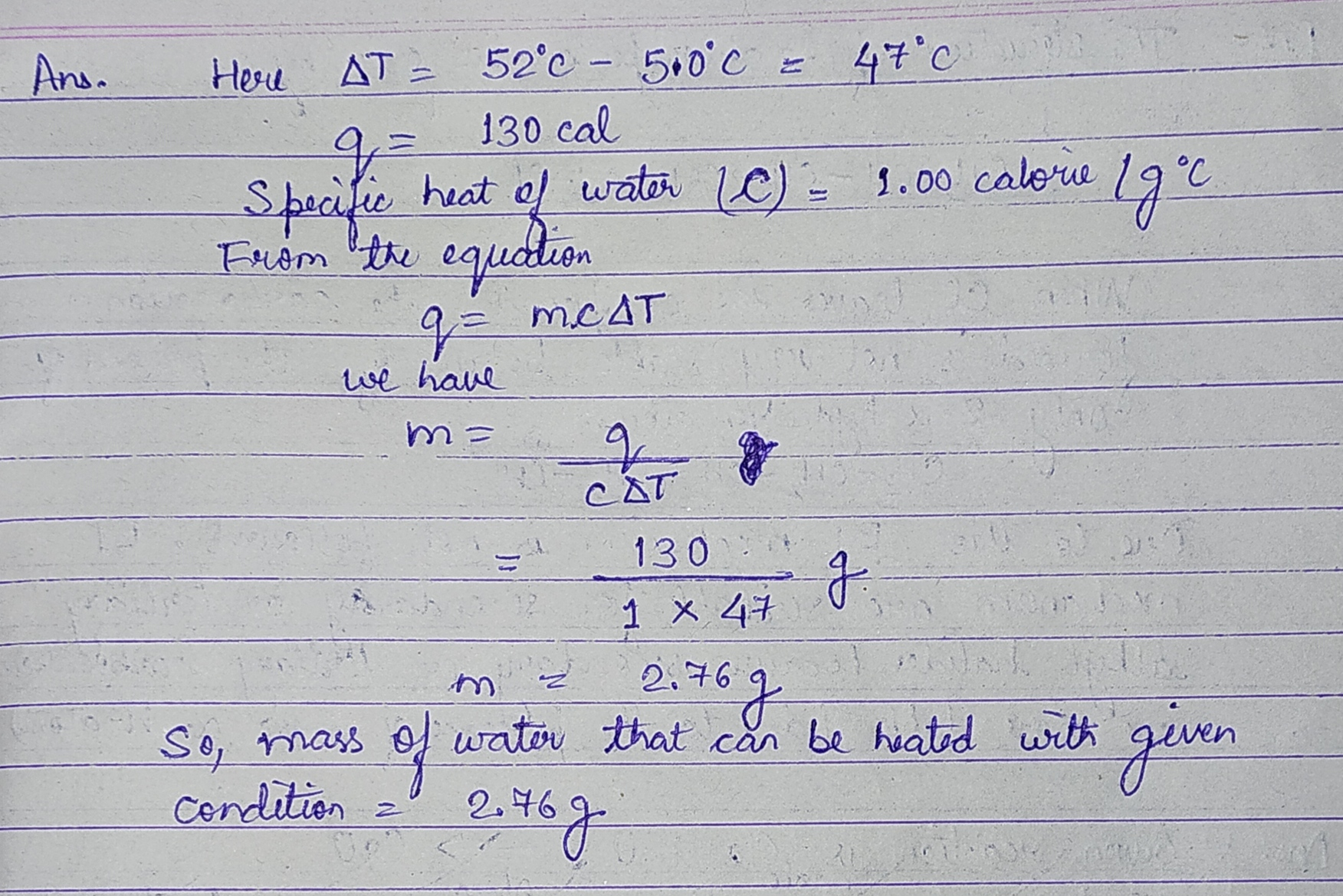#### Earn Coin

Coins can be redeemed for fabulous gifts.

Similar Homework Help Questions
• ### What mass of water can be heated from 5.0 °C to 52 °C with 130 cal...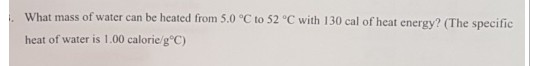What mass of water can be heated from 5.0 °C to 52 °C with 130 cal of heat energy? (The specific heat of water is 1.00 calorie/g°C)

• ### How much heat is released when 15g of ice is heated from -12 °C to water vapor at 105 °C? (AH fus = 79.5 cal/g, AH...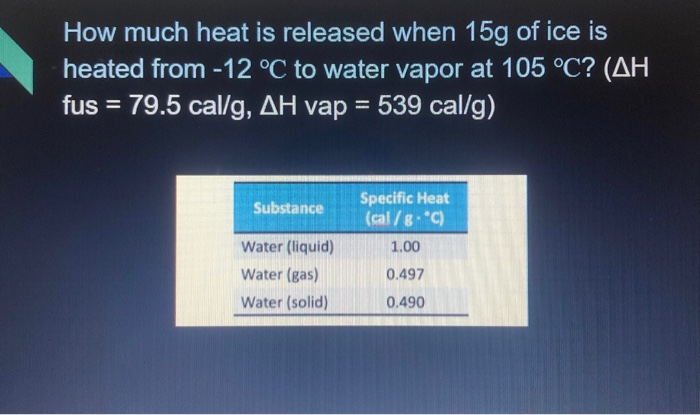How much heat is released when 15g of ice is heated from -12 °C to water vapor at 105 °C? (AH fus = 79.5 cal/g, AH vap = 539 cal/g) Substance Specific Heat (cal/g °C) 1.00 Water (liquid) Water (gas) Water (solid) 0.497 0.490

• ### can someone please help me solve this lab? calorie since it takes 1.00 cal to rai...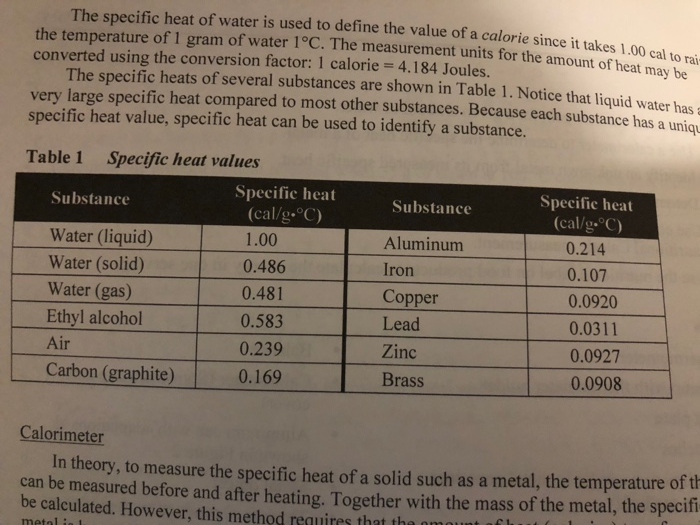can someone please help me solve this lab? calorie since it takes 1.00 cal to rai at units for the amount of heat may be The specific heat of water is used to define the value of a calorie since it takes 1 the temperature of 1 gram of water 1°C. The measurement units for the amount converted using the conversion factor: 1 calorie = 4.184 Joules. The specific heats of several substances are shown in Table 1. Notice that...

• ### Find what heat in calories (cal) is required to increase the temperature of 52 g water...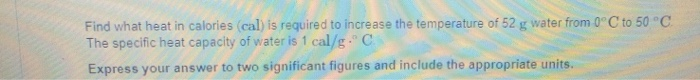Find what heat in calories (cal) is required to increase the temperature of 52 g water from 0°C to 50 °C The specific heat capacity of water is 1 cal/g. C Express your answer to two significant figures and include the appropriate units. Calculate the quantity of heat absorbed by 12 g of water that warms from 30°C to 82 °C. Express your answer to two significant figures and include the appropriate units.

• ### 5.175 g of water was heated from 15 °C to 88 °C. How many kilocalories were...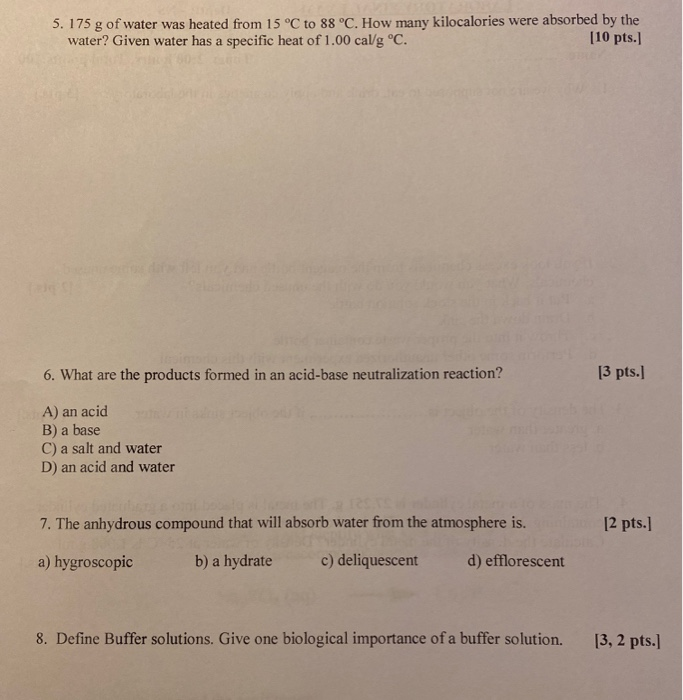5.175 g of water was heated from 15 °C to 88 °C. How many kilocalories were absorbed by the water? Given water has a specific heat of 1.00 cal/g °C. [10 pts.] 6. What are the products formed in an acid-base neutralization reaction? [3 pts.] A) an acid B) a base C) a salt and water D) an acid and water 7. The anhydrous compound that will absorb water from the atmosphere is. [2 pts.] a) hygroscopic b) a hydrate...

• ### A 130 g copper bowl contains 210 g of water, both at 25.0°C. A very hot...

A 130 g copper bowl contains 210 g of water, both at 25.0°C. A very hot 450 g copper cylinder is dropped into the water, causing the water to boil, with 16.9 g being converted to steam. The final temperature of the system is 100°C. Neglect energy transfers with the environment. (a) How much energy is transferred to the water as heat? (b) How much to the bowl? (c) What is the original temperature of the cylinder? The specific heat...

• ### 3 pts Question 11 A 126 gram sample of ice was heated from 11.4°C to 42°C....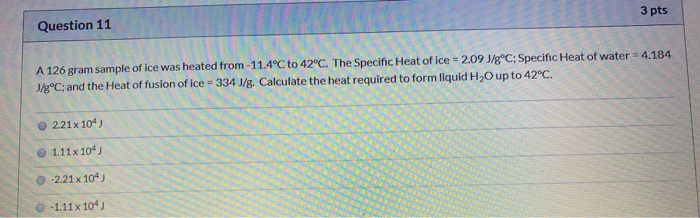3 pts Question 11 A 126 gram sample of ice was heated from 11.4°C to 42°C. The Specific Heat of ice = 2.09 J/gºC; Specific Heat of water = 4.184 J/gºC; and the Heat of fusion of ice = 334 J/g. Calculate the heat required to form liquid H2O up to 42°C. 221x104) 1.11 x 104) -2.21 x 104) -1.11 x 104)

• ### / what mass of water can be heated from 25.0° C to 50.0° C by the...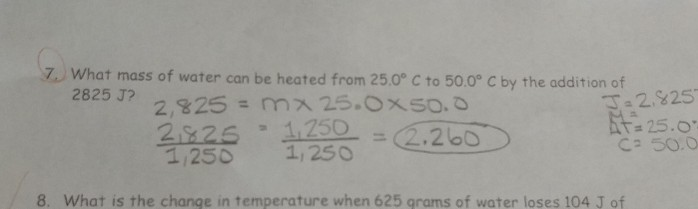/ what mass of water can be heated from 25.0° C to 50.0° C by the addition of 2825 J? 2,825= mㅈ25.oxso. 2826 250.260 1,250 1,250 2, &25 At 25.0 2.2b0 8. What is the change in temperature when 625 grams of water loses 104 J of

• ### ockhart 3 pts Question 12 A 126 gram sample of ice was heated from -11.4°C to...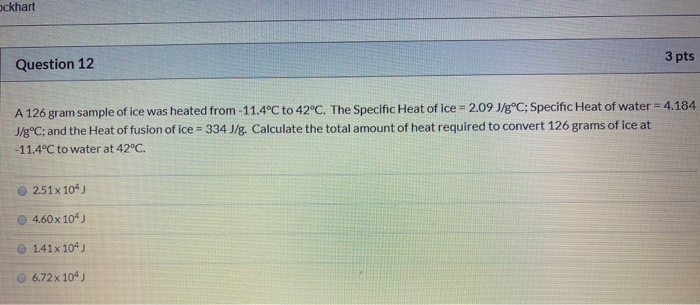ockhart 3 pts Question 12 A 126 gram sample of ice was heated from -11.4°C to 42°C. The Specific Heat of ice = 2.09 J/gºC; Specific Heat of water = 4.184 J/gºC; and the Heat of fusion of ice = 334 J/g. Calculate the total amount of heat required to convert 126 grams of ice at -11.4°C to water at 42°C. 2.51x 104) 4.60 x 104) 1.41x 104 6.72 x 104

• ### A metal object with mass of 20.8 g is heated to 97.0 °C and then transferred to an insulated container containing 8...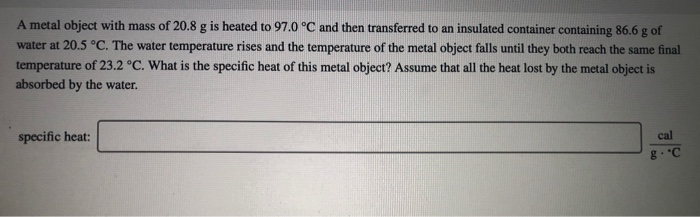A metal object with mass of 20.8 g is heated to 97.0 °C and then transferred to an insulated container containing 86.6 g of water at 20.5 °C. The water temperature rises and the temperature of the metal object falls until they both reach the same final temperature of 23.2 °C. What is the specific heat of this metal object? Assume that all the heat lost by the metal object is absorbed by the water. specific heat: cal